﻿ GEOMETRICAL ISOMERISM | ORGANIC | CIS-TRANS | E-Z NOTATION | ALKENES | OXIMES

STEREOISOMERISM IN ORGANIC COMPOUNDS

The isomerism which arises due to different spatial arrangements of atoms or groups is called stereo isomerism. It is broadly divided into:

The stereo isomers have same structural formula i.e., same connectivity order between atoms. However the atoms or groups are arranged differently in space.

# GEOMETRICAL ISOMERISM

The geometrical isomerism arises when atoms or groups are arranged differently in space due to restricted rotation of a bond or bonds in a molecule.

E.g.

1) Two different spatial arrangements of methyl groups about a double bond in 2-butene give rise to the following geometrical isomers.

i.e., cis-2-butene and trans-2-butene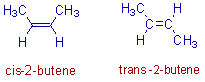Above two forms are not inter convertible due to restricted rotation of double bond.

In the cis isomer, the two methyl groups are arranged on the same side of a double bond. Whereas in the trans isomer, they are on the opposite side.

2) There are two geometrical isomers (cis & trans) possible in case of 1,4–dimethylcyclohexane as shown below: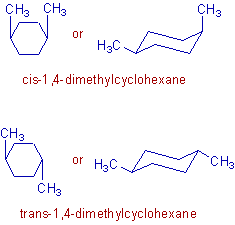In the above geometrical isomers, the methyl groups are arranged differently about the plane of the cyclohexane ring. These isomers are not inter convertible since it is not possible to rotate the bonds in the cyclohexane ring.

* The geometrical isomers often show different physical and chemical properties. The difference in their physical properties is more significant when there is more difference in their polarity.

* Usually the dipole moment of cis-isomers is greater than that of trans isomers. Hence the cis isomers usually have more solubility in polar solvents.

* In general, the trans isomers are more stable than cis isomers.

# E-Z NOTATION

The simple convention of denoting the geometrical isomers by cis/trans descriptors is not sufficient when there are more than two different substituents on a double bond. To differentiate the stereochemistry in them, a new system of nomenclature known as the E-Z notation method is to be adopted.

According to this method, if the groups with higher priorities are present on the opposite sides of the double bond, that isomer is denoted by E.

Where E = Entgegen  ( the German word for 'opposite')

However, if the groups with higher priorities are on the same side of the double bond, that isomer is denoted by Z.

Where Z = Zusammen (the German word for 'together')

The letters E and Z are represented within parentheses and are separated from the rest of the name with a hyphen.

Step by step procedure to determine the E-Z configuration:

The following procedure is to be adopted to denote the geometrical isomers by E & Z descriptors.

* First determine the higher priority group on each end of the double bond.

* If the higher priority groups are on the opposite sides of double bond, the isomer is denoted by the descriptor, E.

* Otherwise if they are on the same side of double bond, the Z descriptor must be used.

The priorities are assigned by following Cahn-Ingold-Prelog sequence rules (CAN rules) described below.

* Rank the atoms directly attached to the olefinic carbon according to their atomic number. High priority is given to the atom with higher atomic number.

* If isotopes of same element are present, the higher priority is given to the isotope with higher atomic mass.

E.g.

The Deuterium isotope (H2 or D) has more priority than protium (H1 or H).

The  C13 isotope has more priority than C12.

* If the atoms are still identical, examine the next atoms along the chain until a “first point of difference” is found. This is done by making a list of atoms linked directly to the first atom. Each list is arranged in order of decreasing atomic number. Then the higher priority is given to the list which contain atom with higher atomic number at first point of difference.

E.g. Examine the lists of atoms directly linked to the highlighted carbons in the following compound, (2Z)-2-tert-Butyl-3-methylpent-2-en-1-ol.The (C,H,H) list has more priority over (H,H,H). Whereas, the (O,H,H) has more priority over (C,C,C). Since the groups with highest priorities are on the same side of the double bond, the descriptor, Z is used to represent the stereochemistry of groups at double bond.

*The multiple bonds are counted as multiples of that same atom i.e., each π bond is treated as if it were another σ bond to that type of atom.

E.g.Illustrations:

1) The cis and trans 2-butenes can also be represented as Z and E isomers respectively.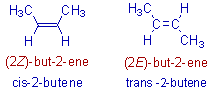Since the double bond is at 2nd carbon of the butene, the number 2 is used before the descriptors i.e., '2Z' or '2E' .

2) There are four geometrical isomers possible with 3-methylhexa-2,4-diene as shown below. The geometries at each double bond are clearly mentioned by the numbers: 2 and 4.3) If the double bond has two similar groups on the same carbon, then there is no need of descriptor. For example, in the following compound, 2,3,4-trimethylhexa-2,4-diene, the double bond on 1st carbon has two methyl groups. Hence there is no need of the descriptor for this double bond.# GEOMETRICAL ISOMERISM IN OXIMES

The oximes are formed when carbonyl compounds are treated with hydroxyl amine. These are represented as: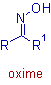Where R & R1 are hydrogens; or alkyl or aryl groups.

The oximes are of two types:

1) Aldoximes: These are derived from aldehydes. In this case, at least either R or R1 is hydrogen.

2) Ketoximes: These are derived from ketones. In this case, both R or R1are alkyl or aryl groups only.

The oximes show geometrical isomerism due to restricted rotation of C=N bond. Two geometrical forms are possible for the oximes as shown below.The descriptors, syn and anti are used to distinguish them.

* In case of aldoximes, the syn form is the one in which both the hydrogen and the hydroxyl (-OH) group are on the same side of the C=N. Whereas in the anti form, they are on the opposite side.

E.g. The syn and anti forms of acetaldoxime are shown below.* However with ketoximes, the syn and anti descriptors indicate the spatial relationship between the first group cited in the name and the hydroxyl group. For example, the following ketoxime of butanone can be named as either syn methyl ethyl ketoxime or anti ethyl methyl ketoxime.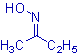E-Z notation of oximes:

The geometrical isomers are better differentiated by using E-Z notations. The Z oxime has hydroxyl group and the group with higher priority on the same side of C=N. However in the E oxime, they are arranged on the opposite sides of the C=N.

E.g. The syn acetaldoxime is named as (E)-acetaldoxime, since the hydroxy group and the group with higher priority i.e., methyl group are on the different sides of the C=N. Whereas the anti form is named as (Z)-acetaldoxime.

IUPAC SYSTEM OF NOMENCLATURE OF OXIMES:

* In the IUPAC system of nomenclature, the oximes are named as: N-hydroxyalkanimines, where the C=N group is represented by the suffix, imine and the -OH group on nitrogen is represented by the prefix, N-hydroxy.

E.g. The syn and anti forms of acetaldoxime are named as follows: